# Mathematical Functions - Excel 2013

## Introduction

A very important feature in Excel is the formula. It is used to calculate values based on what is in cells, perform operations on a cell content, fetch values after an operation based on your search criteria and much more.

Mathematical Formulas in Excel are used to perform various arithmetic operations like sum, average, count, max, min etc. Here is a list of most frequently used mathematical formulas in excel.

SUM():

This function is used to adds all the values within a cell range.

Syntax:

```sum(cell address : cell address)
```

Example: sum(C1:C3)=15

Here in the example below, we will create a basic function to calculate the sum of working hours generates in a day.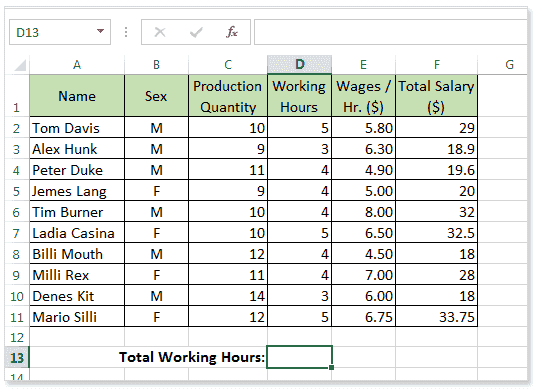Select the cell where you want to put the formula, type the equals sign (=) and write the desired function name or choose the function from the suggested function list. Here in the example below we write the SUM function.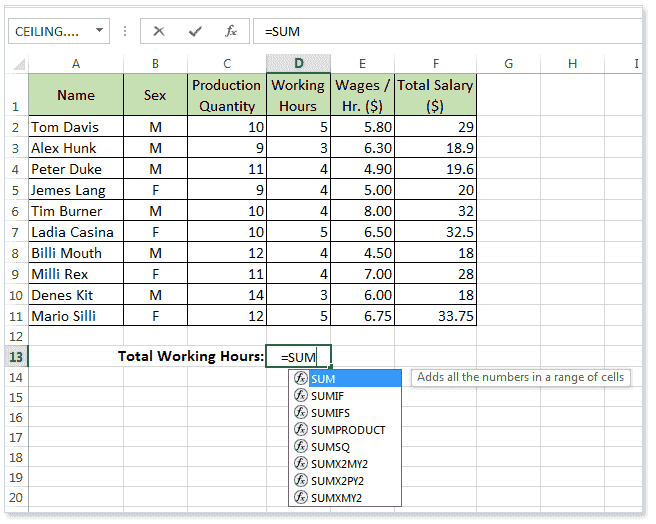Now write the range of sum or you can select the range by using the mouse to drag.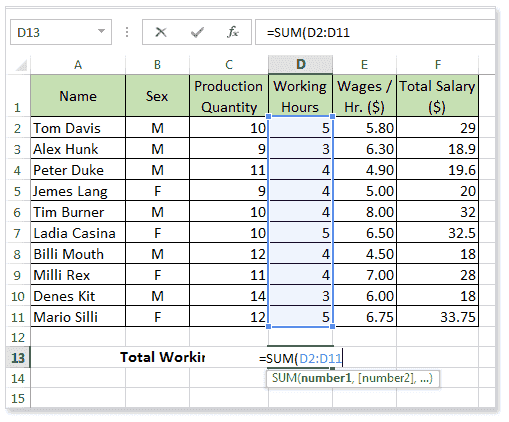Now press Enter key to see the result or press Ctrl+Enter key to stay in the formula cell. Here is the picture below.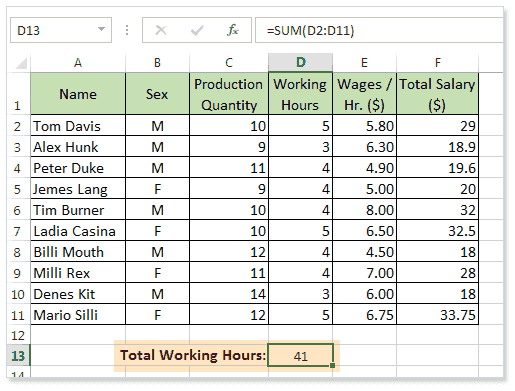You can use the sum() function in other ways. Here is the syntax.

sum(number1,number2,number3....)

Example: sum(4,5,6)=15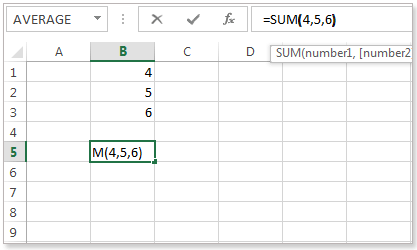SUMIF():

Here in the example below, we will create a basic function to calculate the sum of working hours generates in a day only for female employees.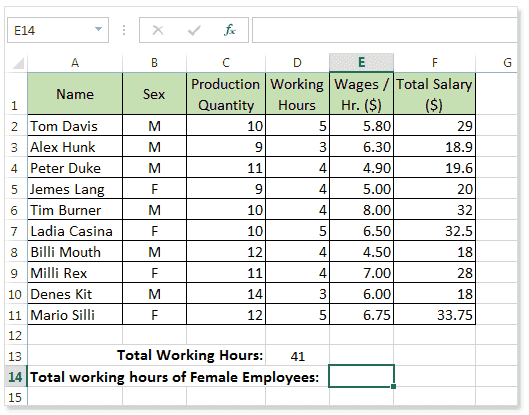Syntax:

```SUMIF(  range,criteria)
```

Type the equals sign and write the desired function in the cell E14. Here is the picture below.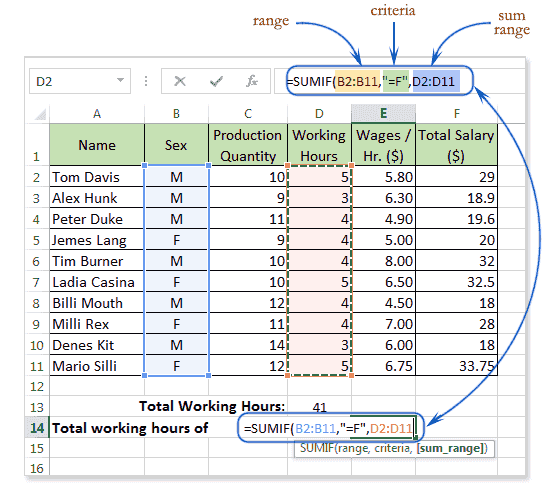Press Enter to see the result and move the cell pointer to below cell or press Ctrl+Enter to stay on the cell.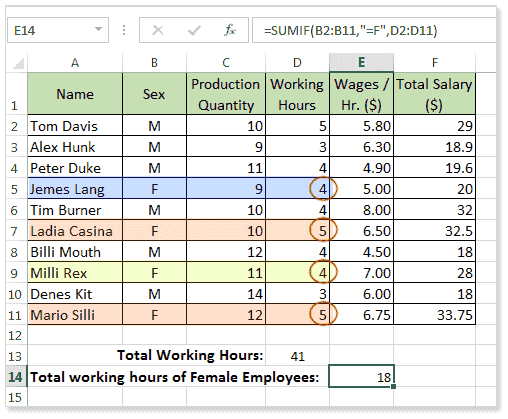AVERAGE():

Here in the example below, we will create a basic function to calculate the average working hours of each employee.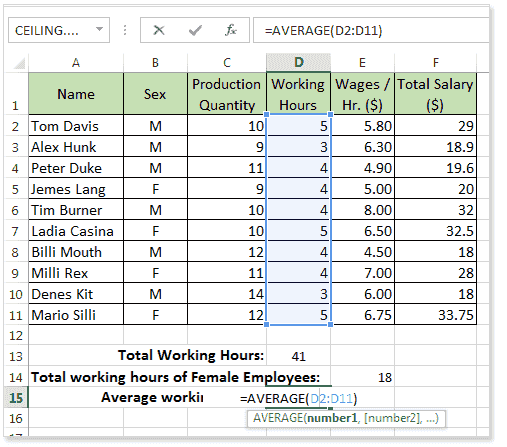Press Enter key and see the result.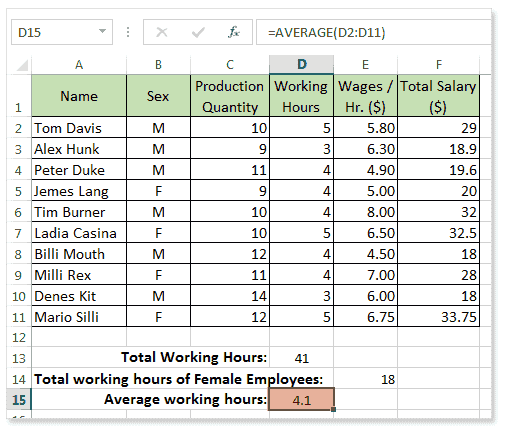You can use the AVERAGEIF() and AVERAGEIFS() function in a similar way as SUMIF() function, to average cells based on one or multiple criteria.

COUNT()

Here in the example below, we will create a basic function to calculate the number of employees.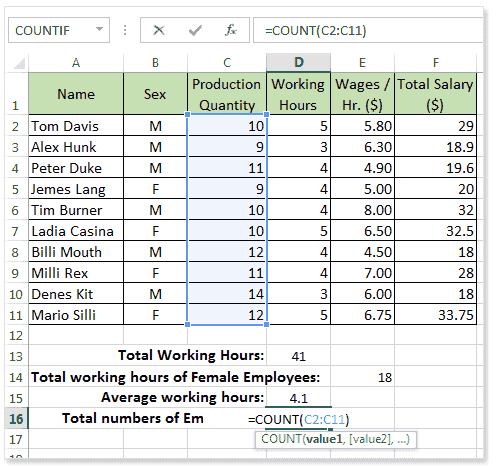ROUND():

The round function is used to round a number to a specified number of digits.

Syntax:

```ROUND(number, number_of_digits)
```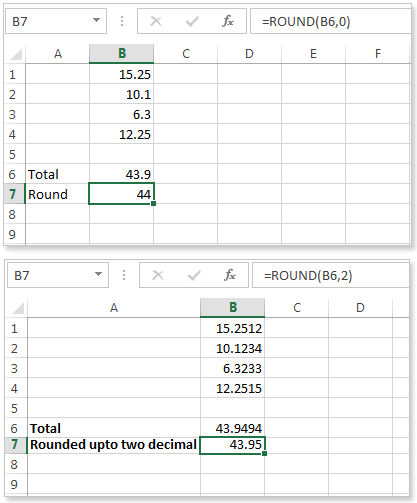RAND():

This function is used to returns a random number greater than or equal to 0 and less than 1.

Syntax:

```RAND()
```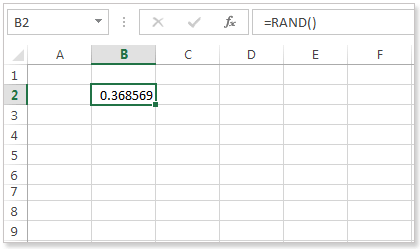MOD()

This function is used to find the remainder after dividing a number by another number.

Syntax:

```MOD(number,divisor)
```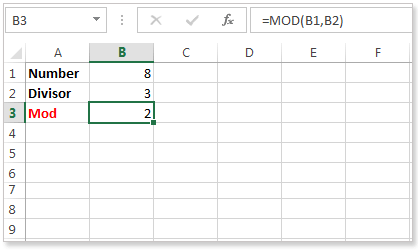INT():

This function is used to converts a decimal number to integer lower than it.

Syntax:

```INT(decimal number)
```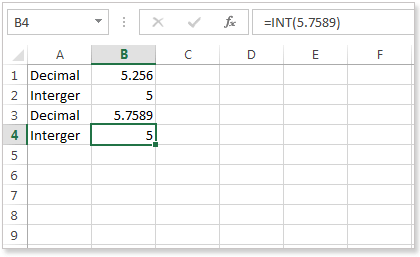AVERAGE():

Formula:

This function is used to calculate the average of a range of cells.

Syntax:

```AVERAGE(number1,number2,.......)
```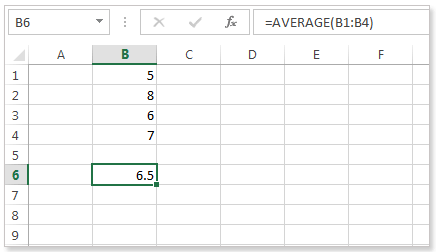ABS():

The abs() function is used to return the absolute value of a given number. The number may be positive or negative. Here is the example below.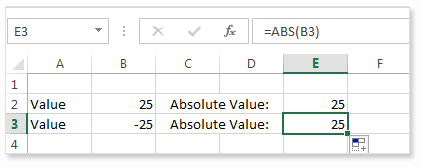ARABIC():

This function is used to convert roman numeral to arabic. This function accepts roman numeral as an argument. The picture below shows that you can write the formula in any cell or you can use the function wizard or you can select any cell and write the formula in the formula bar and press Ctrl+enter to stay the cell or press enter see the result.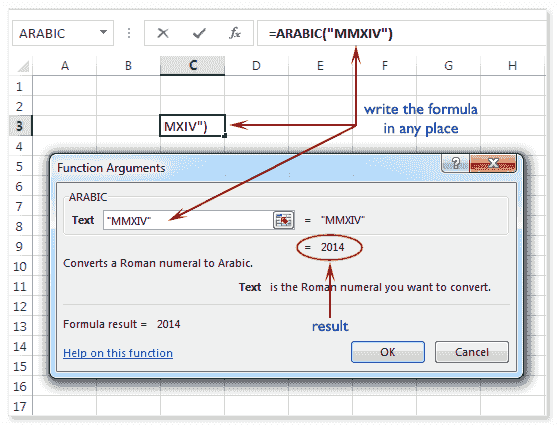CEILING.MATH():

This function is used to rounds a number upto the nearest integer or to the nearest multiple significance. This function accepts three arguments, these are number, significance and mode. Number is a number, significance is the multiple to which you want to round and mode is also a number. Here in the example below the number is 6.423 and the significance is 3 and the nearest multiple of 3 of the given number is 9 and the mode is a nonzero, so this function starts rounding away from zero.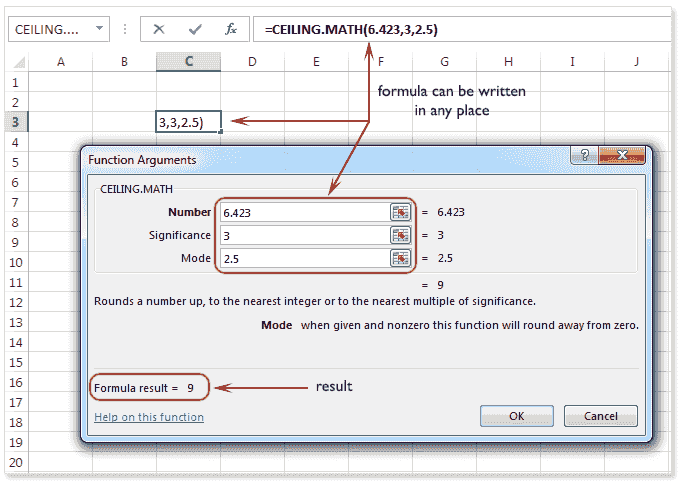Previous: Functions Basic - Excel 2013
Next: Excel BETA.DIST() function

﻿